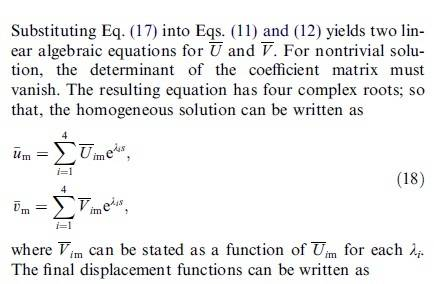# How to get roots of this complex equation

pm1366

## Homework Statement

hello, i am stucked at an article from sciencedirect . somewhere it gives me the following equation and then it tells that this equation must have 4 complex roots!

the variable is lambda and we want to find 4 lambda complex roots

λ^4=0

## The Attempt at a Solution

i am amazed how we can get 4 complex roots from this equation as it seems to have roots equal to zero !

Any help would be greatly appreciated!

## Answers and Replies

Gold Member
The four roots are:

0∠0°
0∠90°
0∠180°
0∠270°

pm1366
The four roots are:

0∠0°
0∠90°
0∠180°
0∠270°

thanks for reply but i forgot to explain more, here it is:

we have 3 linear partial differential equations:

http://i45.fastpic.ru/big/2012/1027/8f/429c9d2a3bd454656212ed589156f38f.jpg

now for homogeneous solution we're going to substitute below Equation into Eqs. (11) and (12) at the above picture:

http://i44.fastpic.ru/big/2012/1027/e0/ef375cf97523a4d907b0d504bd9664e0.jpg

the rest is explained below:now what i've done is substituting Equation 17 into Eqs. (11) and (12), the result is these 2 equations ("s" same as "x" ):

http://i45.fastpic.ru/big/2012/1027/86/b87473ecf6018ce0ab166cfb71a4c086.jpg

don't mind what the coefficients A,D & B are... they're constants.
also L differential operators are given in Appendix and i've used them

now the determinant of the coefficient matrix must vanish (=0) , the result would be this way:

http://i44.fastpic.ru/big/2012/1027/1a/e6d25171f9daaf47e13b1197ff7bf81a.jpg

and in this equation we must find 4 complex roots , i mean 4 complex lambda's
( maybe we can think this equation is correct for all values of x , so we may only have λ^4=0 )

i think your answers are not applicable here

Big thanks to anyOne help me in this matter....

Last edited:
Homework Helper
Yes, obviously the only root is $\lambda= 0$. Since you now tell us that this is the characteristic equation of a differential equation with constant coefficients, that means that four independent solutions to the equation are e0x= 1, x, x2, and x3.

pm1366
Yes, obviously the only root is $\lambda= 0$. Since you now tell us that this is the characteristic equation of a differential equation with constant coefficients, that means that four independent solutions to the equation are e0x= 1, x, x2, and x3.

thanks...this is some likely answer , but here in this article we need 4 different lambda's .
besides, as u see the four independent solutions are different from those u mentioned...that is exponential in the series form and 4 lambda's are included:

http://i45.fastpic.ru/big/2012/1027/2f/678839cd8c87271c8453274f1a30b72f.jpg

aralbrec
That statement is assuming the roots are different. See if you can find something in the article talking about repeated roots and what happens for those.

pm1366
That statement is assuming the roots are different. See if you can find something in the article talking about repeated roots and what happens for those.

no,no where talking about that ... it is an article about cylindrical shells , i can give it to anyone who want to have a look...

aralbrec
no,no where talking about that ... it is an article about cylindrical shells , i can give it to anyone who want to have a look...

Look up 'repeated roots' and 'differential equations' in your textbooks or on the internet.

The solution strategy is this:

You have a homogeneous differential equation (ie one that solves Dy = 0, where D is some differential operator).

Taking a function and adding derivatives of itself to get zero is only possible with the exponential function, so it is assumed that the solution will be of the form y=eλt . Plug that into the differential equation and you have conditions on λ for that solution to work. The condition on λ is called the characteristic equation . Since y=eλt for a specific λ satisfying the characteristic equation has been found to solve the homogeneous equation, a sum of all eλt with every λ found must also solve the homogeneous equation because differentiation is linear. So the total homogeneous solution is Ʃ Aieλit as your excerpt states.

From other considerations we know an nth order differential equation has n independent functions that satisfy the homogeneous equation. If some of the λ are repeated (eg you have four zeroes for λ), you are not finding all those functions so you have to have another way to find them. That part the article hasn't mentioned but HallsOfIvy gave you the solutions -- the idea is to assume the solution is the form of eλt, teλt, t2eλt, ... the highest power of t depending on how many repeated roots you have (if you have four repeated roots, you need four equations with that root). You can confirm these also satisfy the differential equation by substitution and the resulting complete solution will be a summation of all such functions you found.

The idea of doing this is called 'variation of parameters'. The notion was Aeλt is one solution, what if A was allowed to be a function of time, would that work? Plug into the differential equation, and lo and behold, it does with A=tn for some n.

Last edited:
pm1366
@aralbrec
thank you for your help, i get what you're saying , but please look at this red line i've drawn in this picture:
http://i45.fastpic.ru/big/2012/1027/2f/678839cd8c87271c8453274f1a30b72f.jpg

it is showing that there must be 4 different lambda for the solution and not repeated lambda's ( λ i=1,2,3,4 )
in this picture there's no solution for repeated roots...
i mean we must have 4 different roots , but the problem is how to find four different complex roots!

suppose that we know lambda is complex from the beginning ,now how to find 4 complex roots from λ^4=0 or similarly Z^4=0 assuming Z::complex ????? is there any kind of solution except zero?

Last edited:
pm1366
any other ideas friends ????????